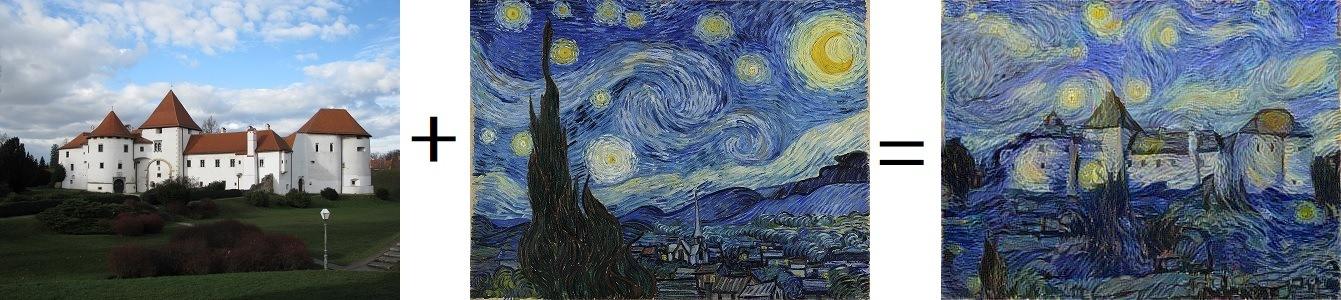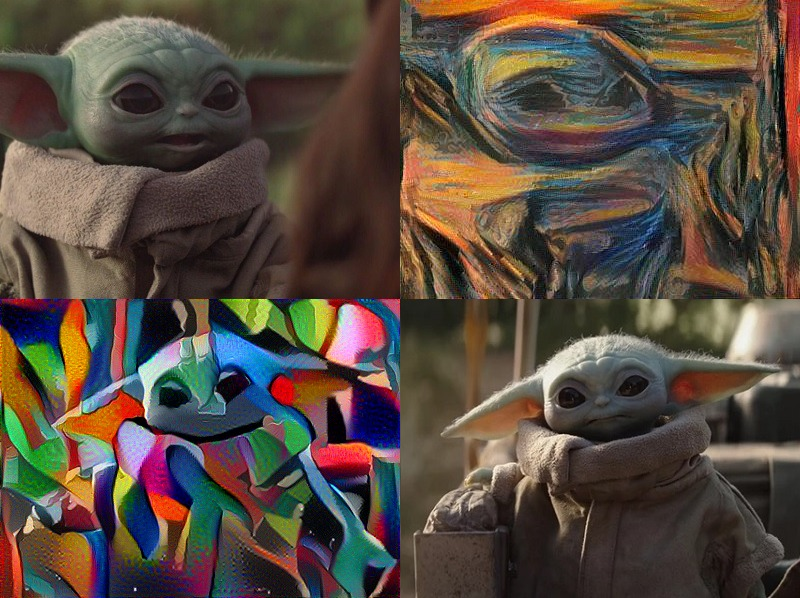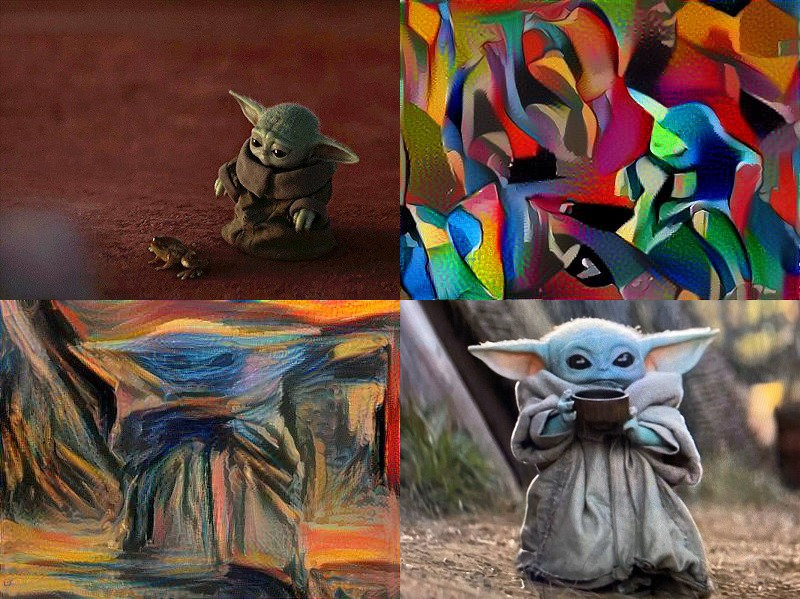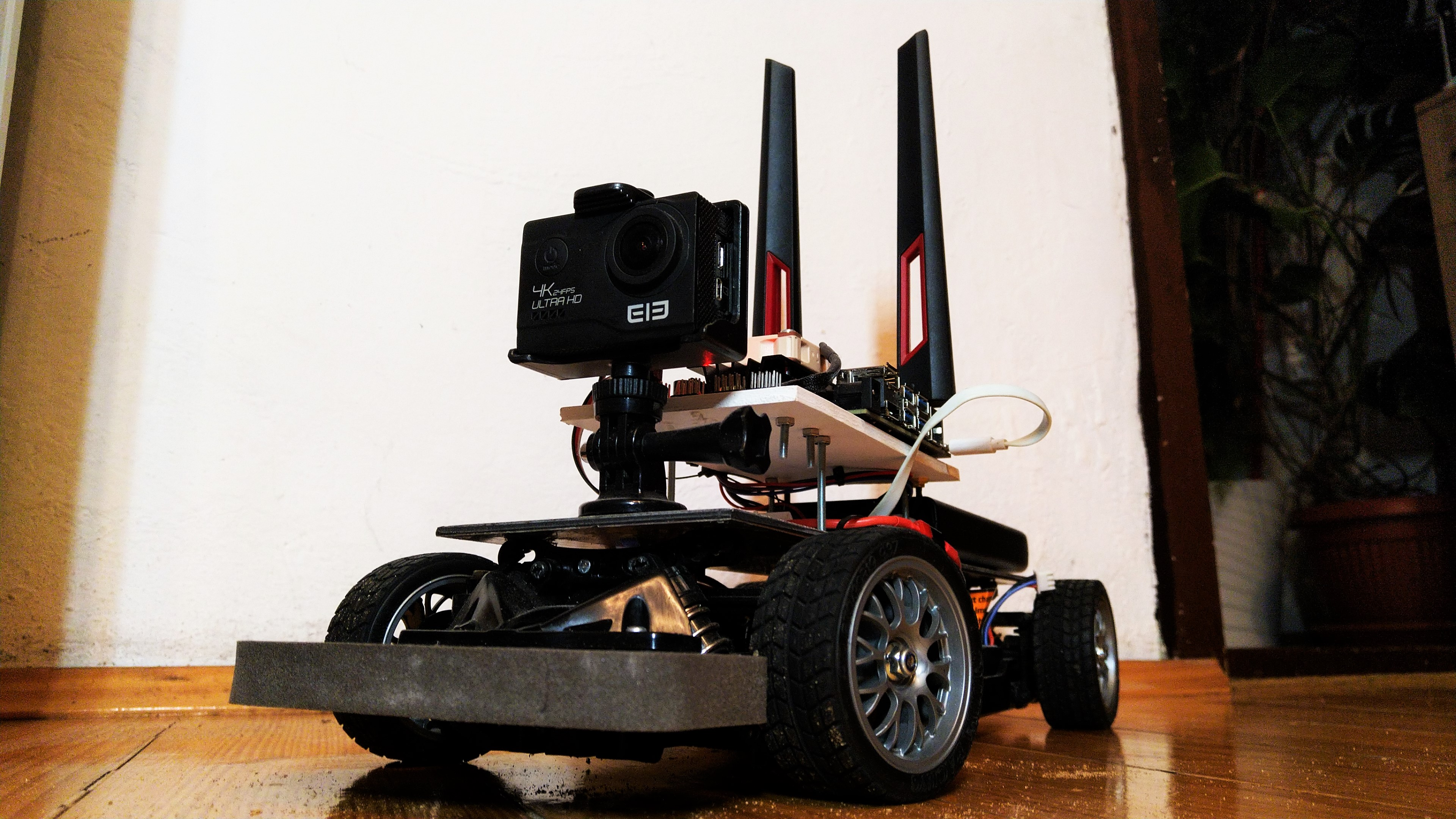# Self-Driving RC Car

Ivan Oršolić - Master's Thesis.

#### Why a self-driving RC car?

🛈 Video source: Waymo, Sensor Visualization

## Bill of materials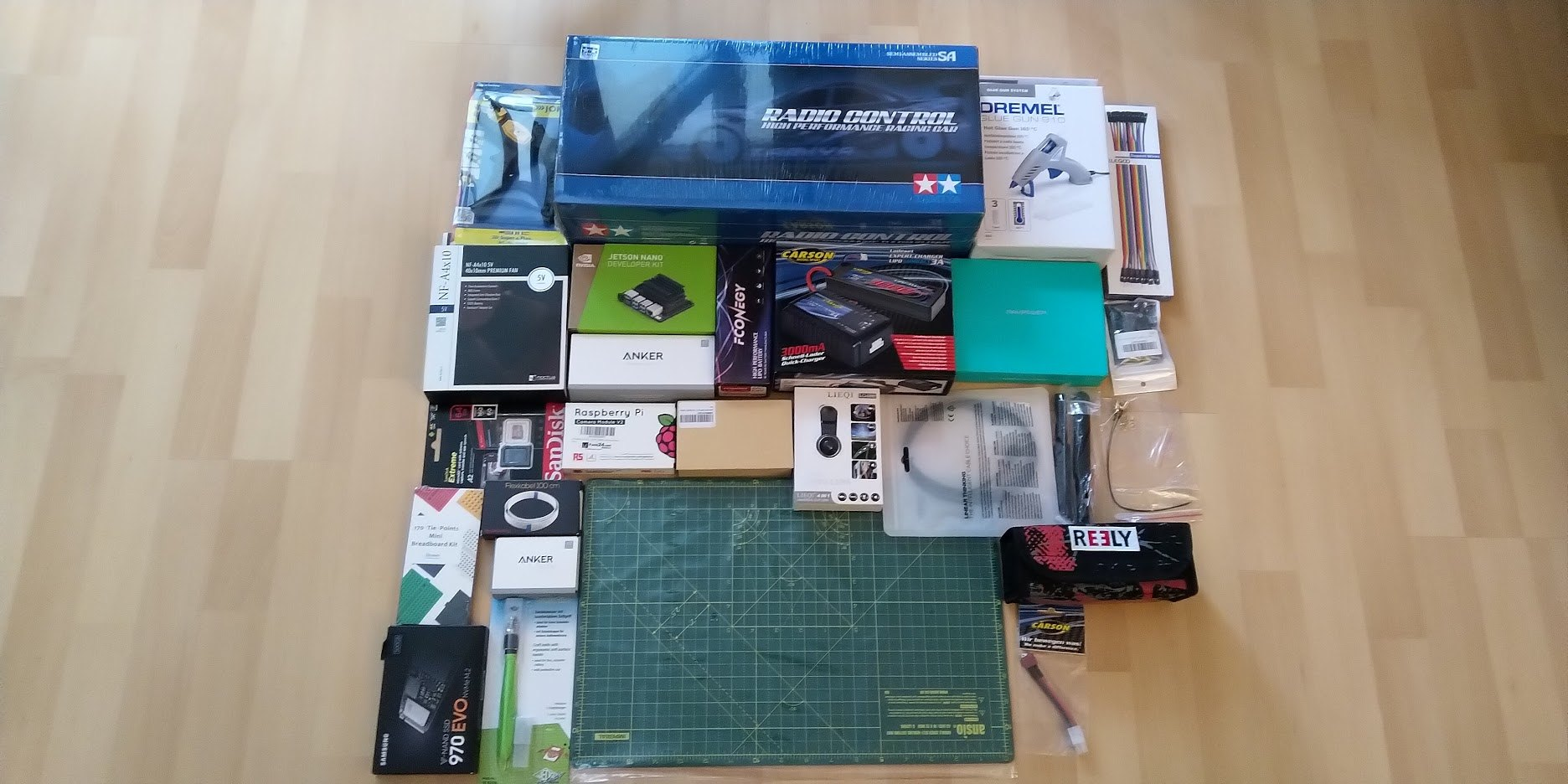## An RC car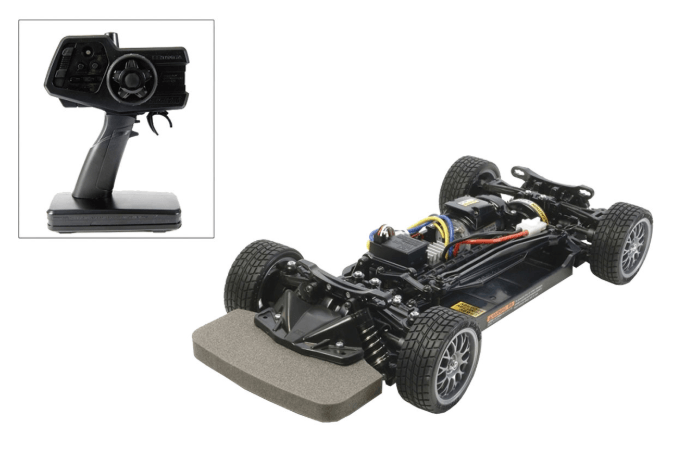## Charger and batteries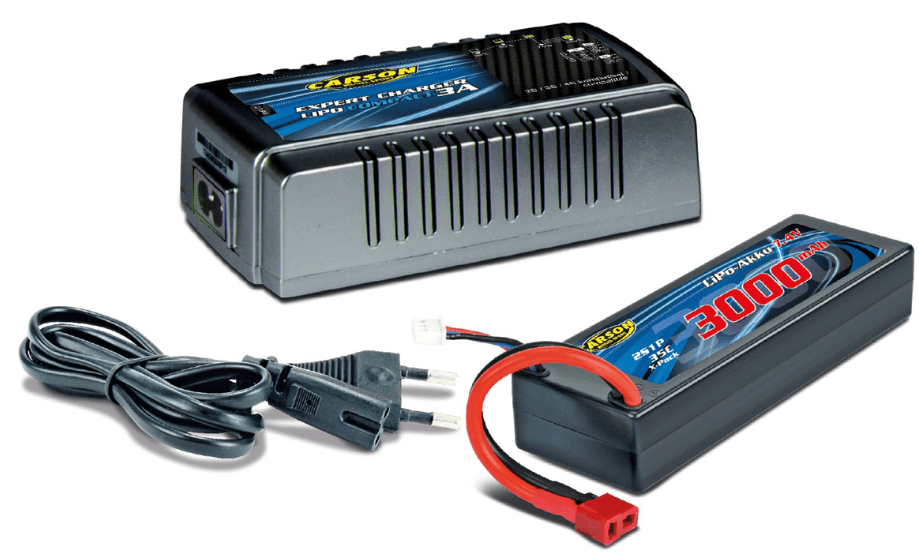## A PWM/Servo Driver (I2C + some jumper cables)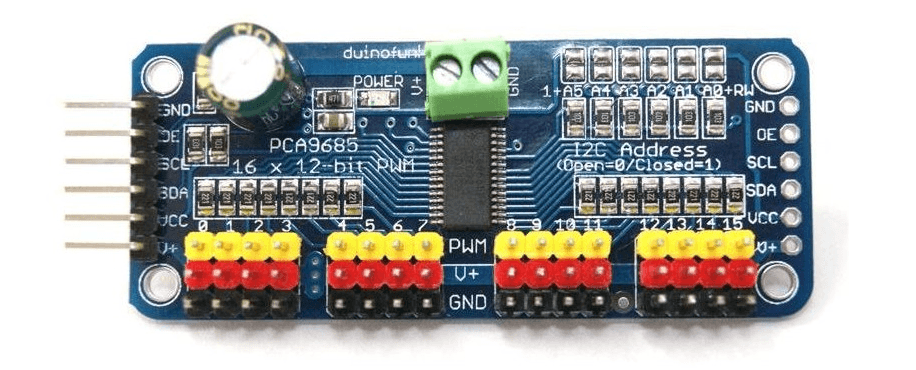## A Jetson Nano## A powerbank (+ some usb cables)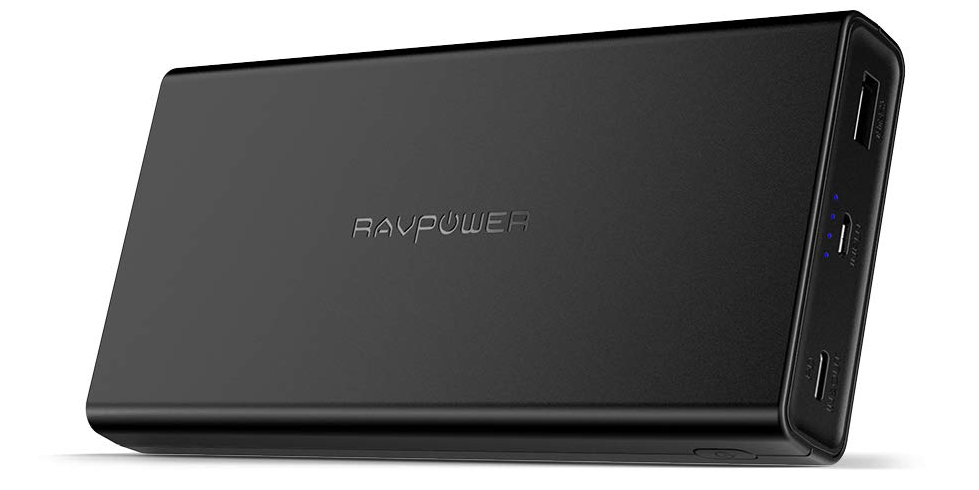## A microSD card (and optionally an external SSD)## A WiFi/BT m.2 card (key E) or some USB equivalent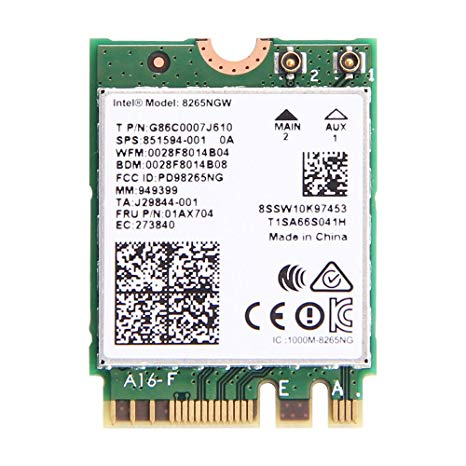## A camera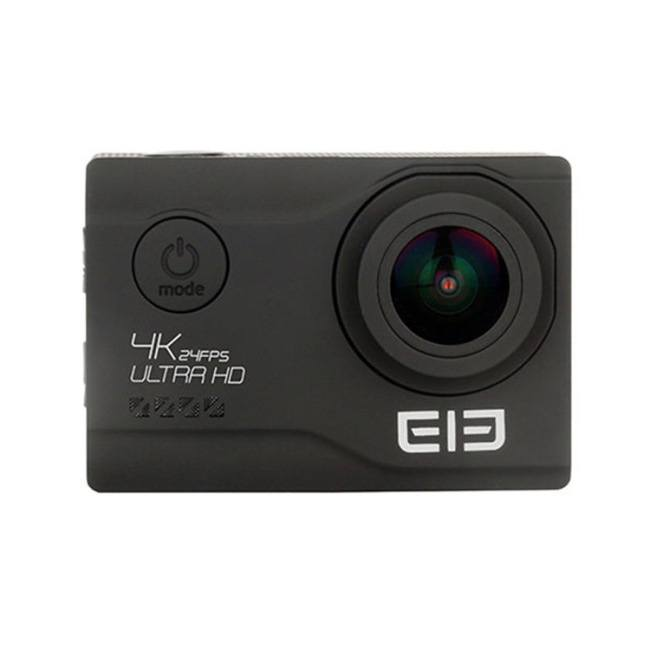### RTR Kit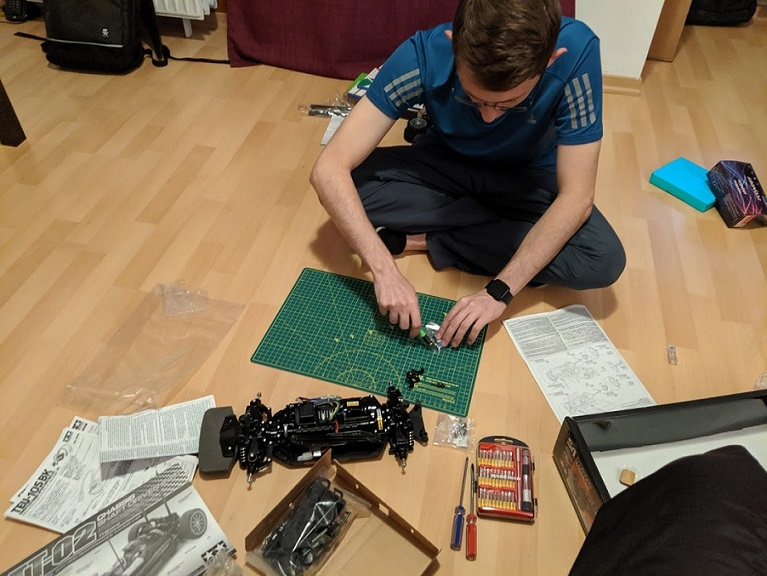### Wheel base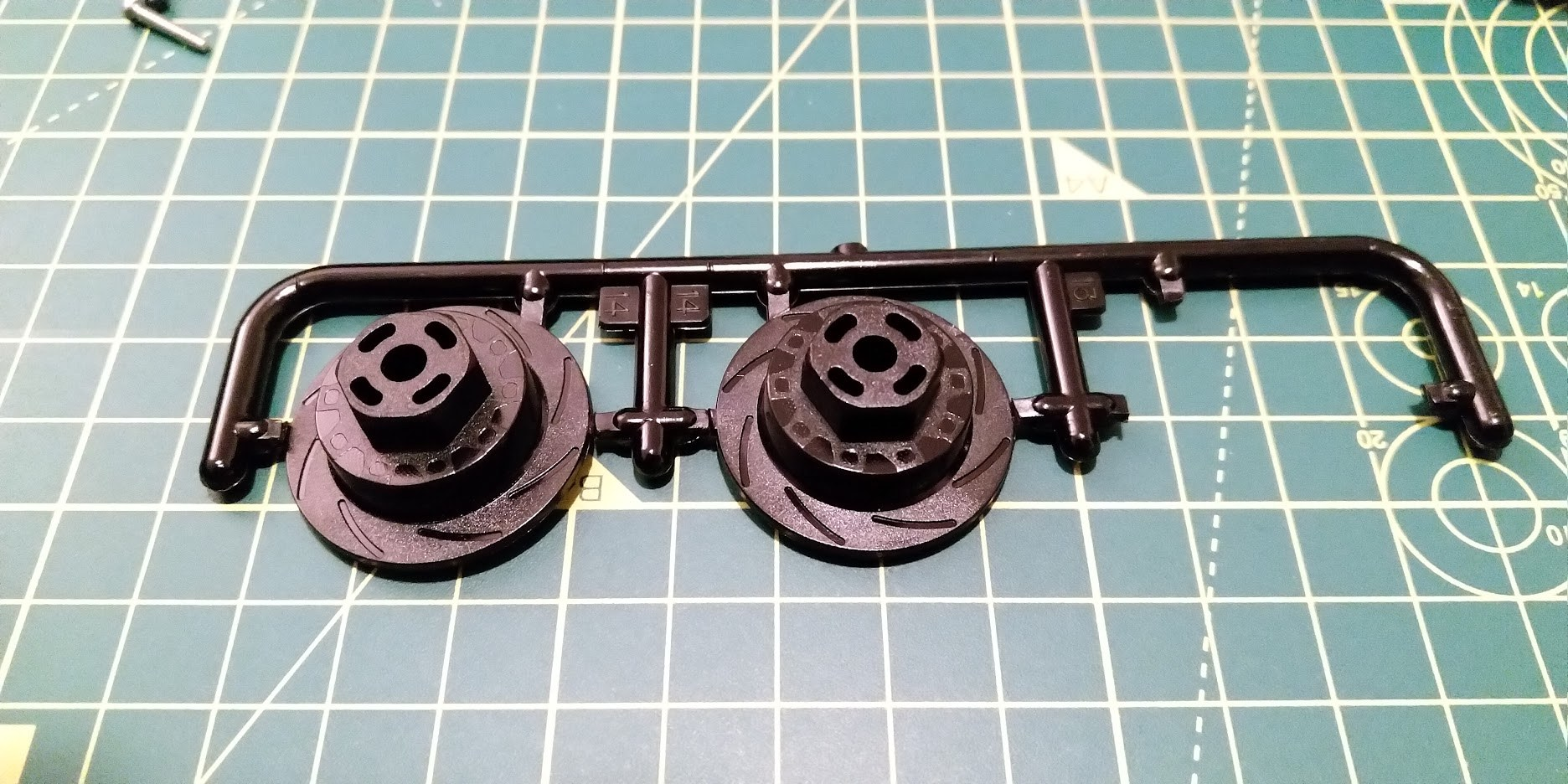### Mounting the wheels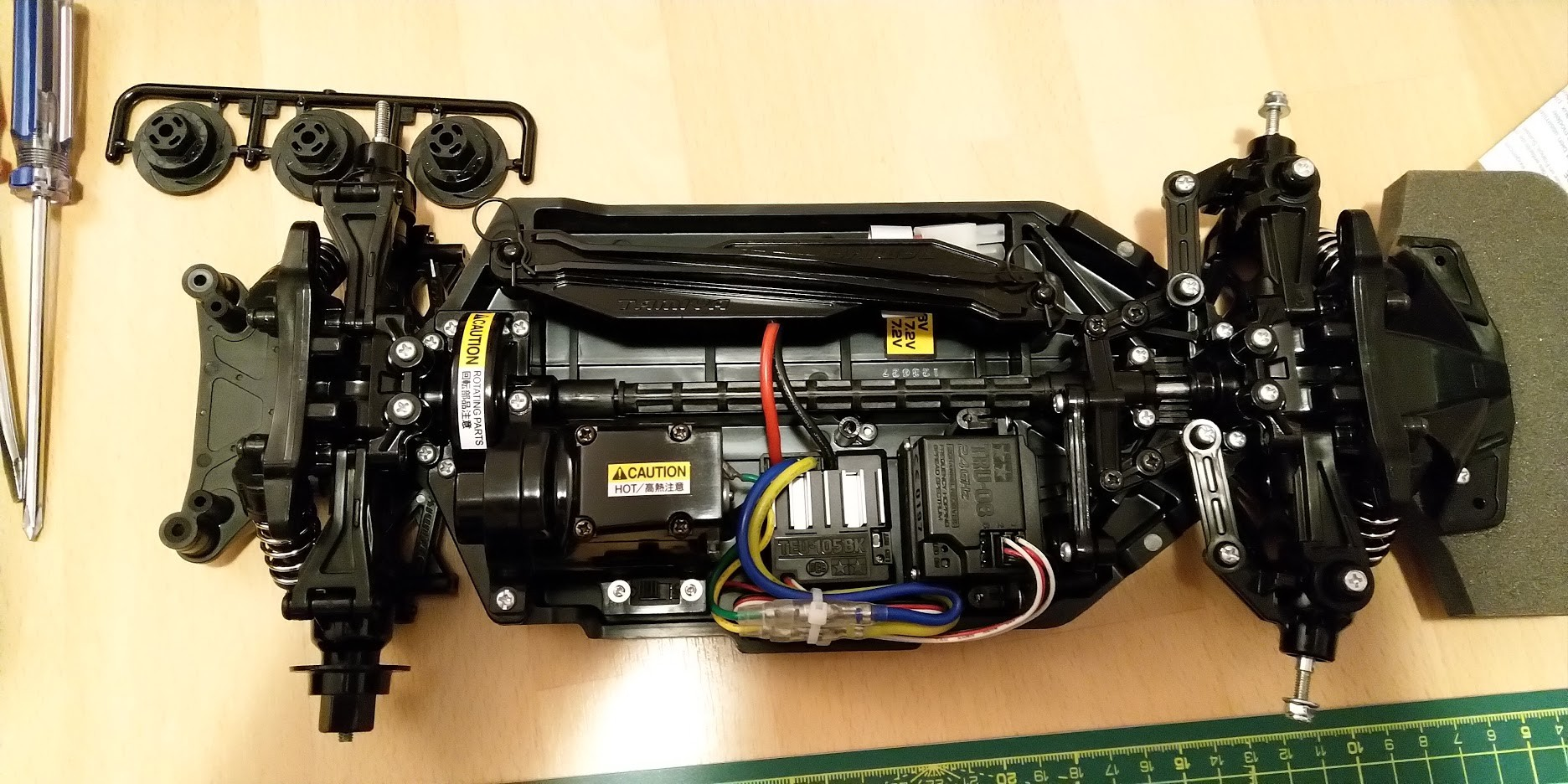### Mounting the wheels## Assembling the mounting plates

### Option 1: 3D Printed parts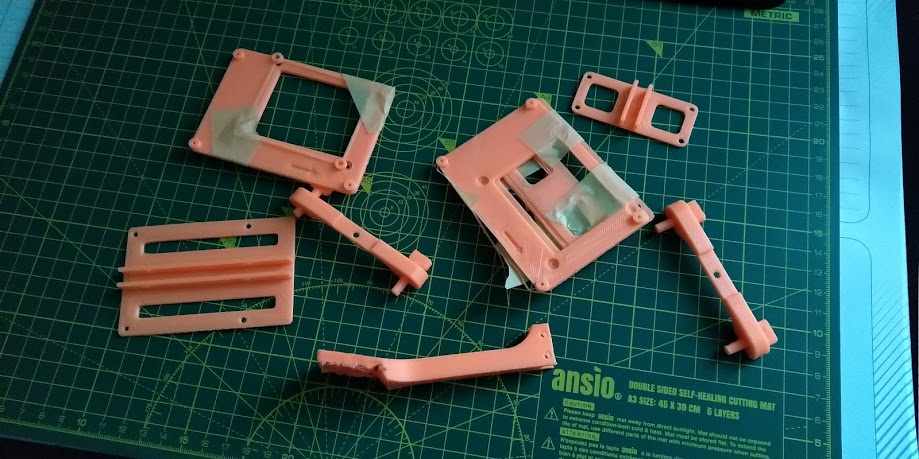### Option 2: Custom made mounting plates### Power tools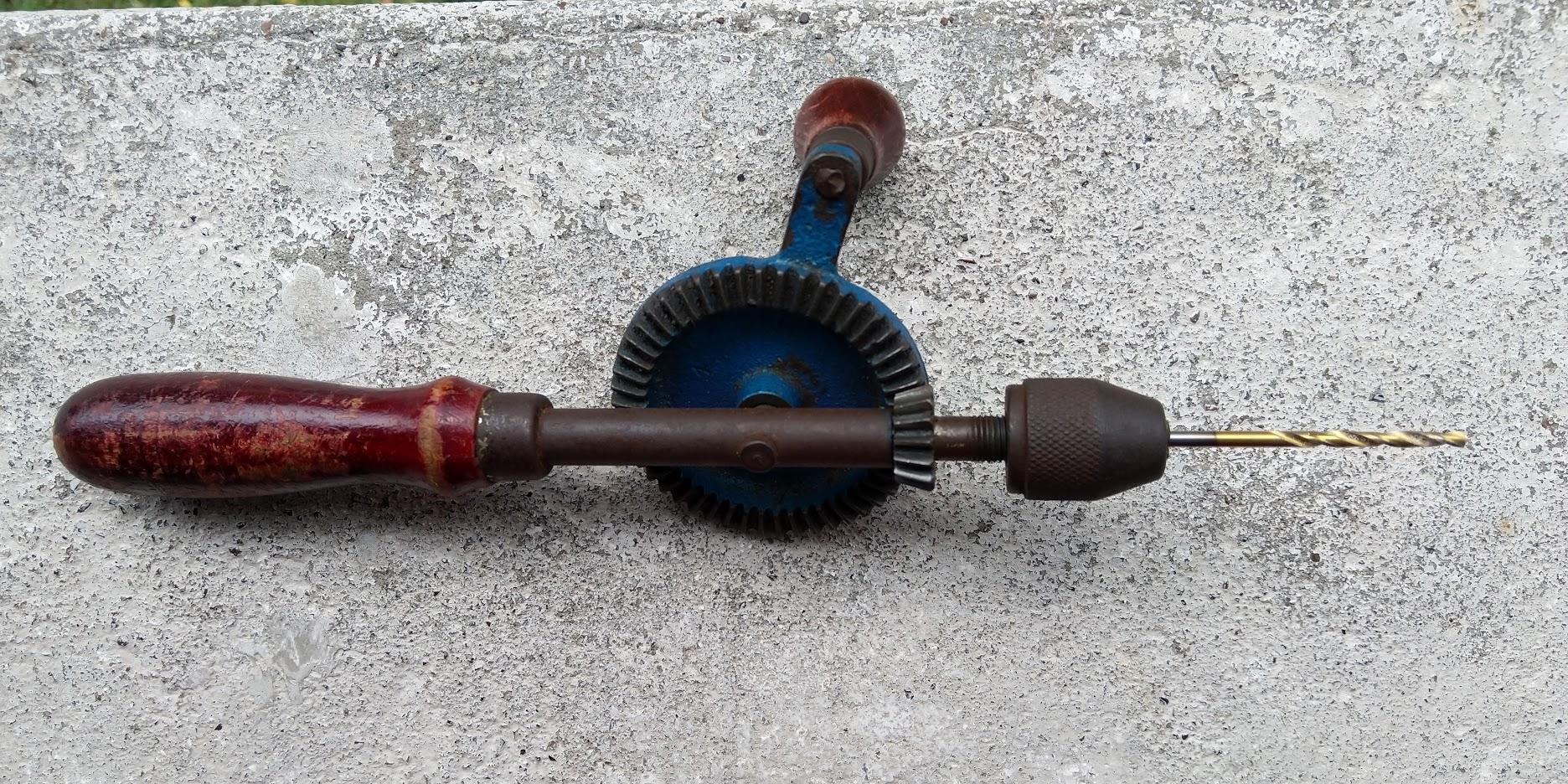### Drilled mounting plates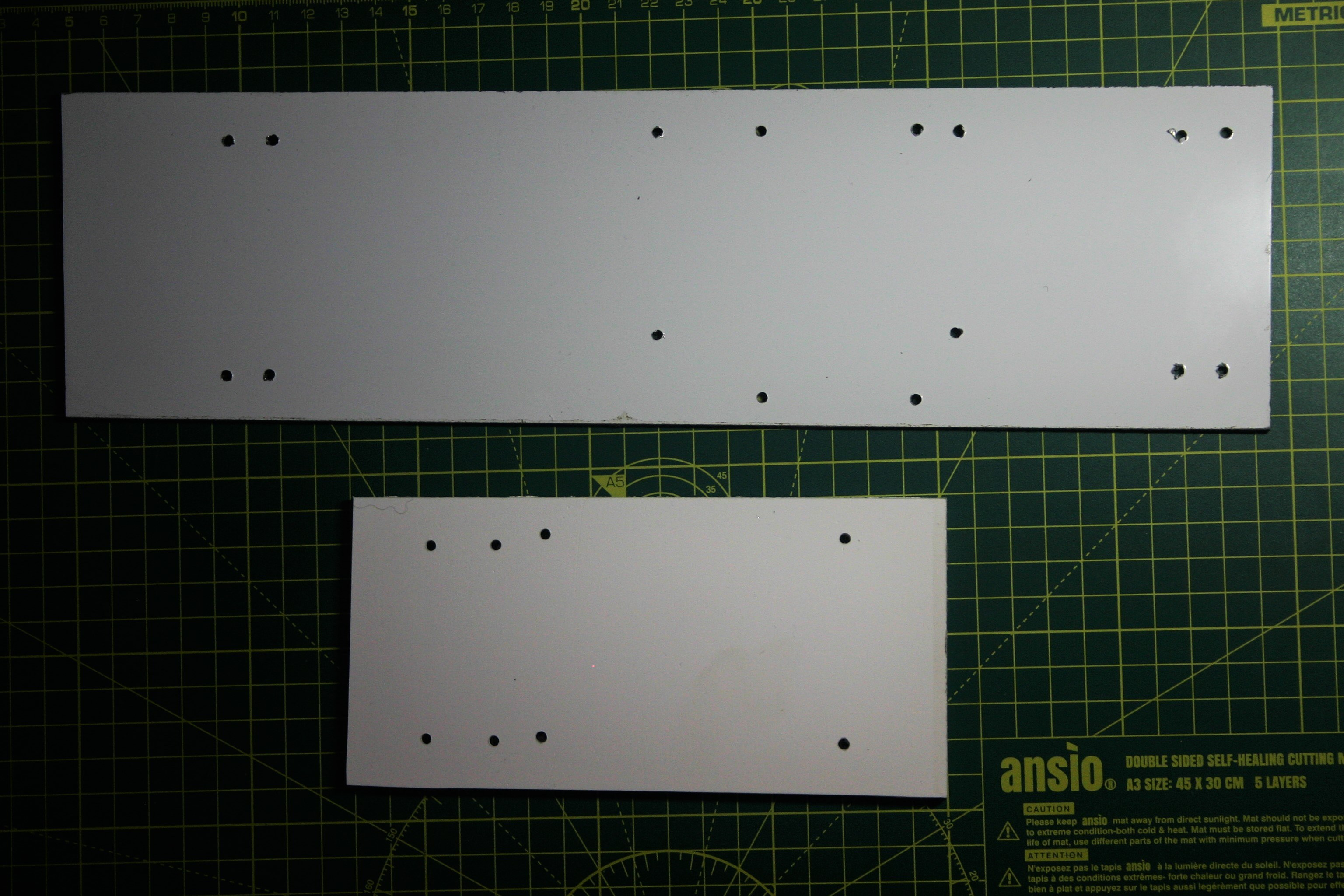### (Semi) Finished car## Jetson Nano assembly

### Taking out the Nano module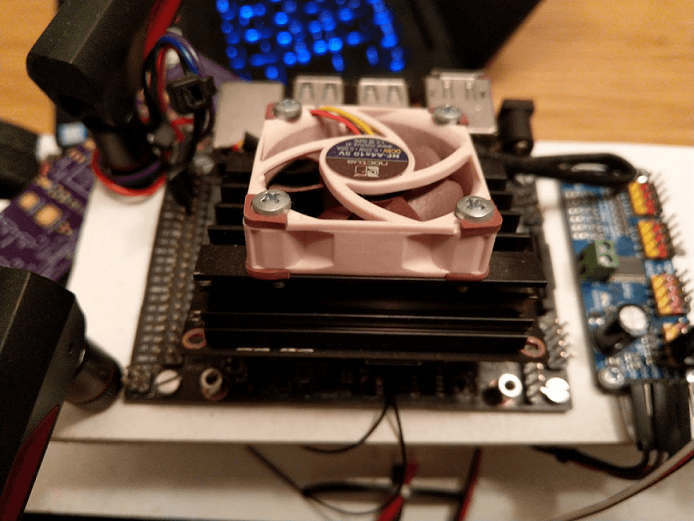### MicroSD slot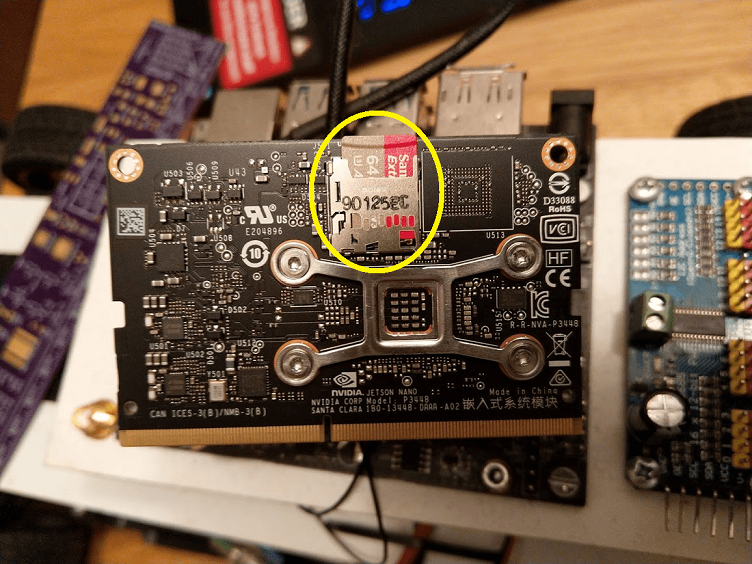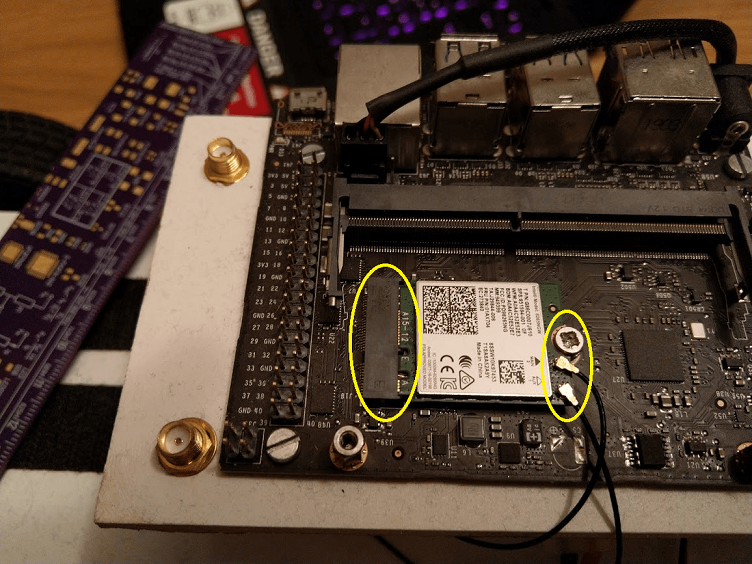## Connecting the RC car to the Jetson Nano

### The schematic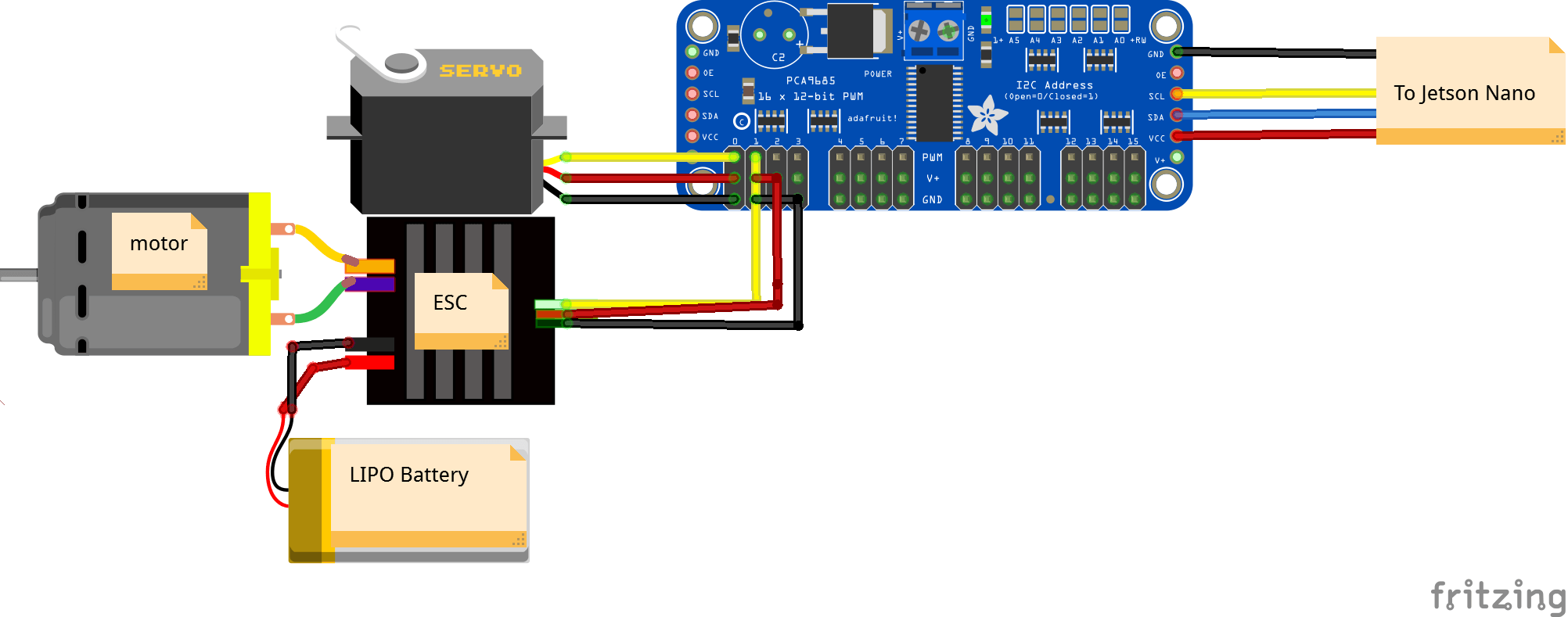### Finding the ESC/Servo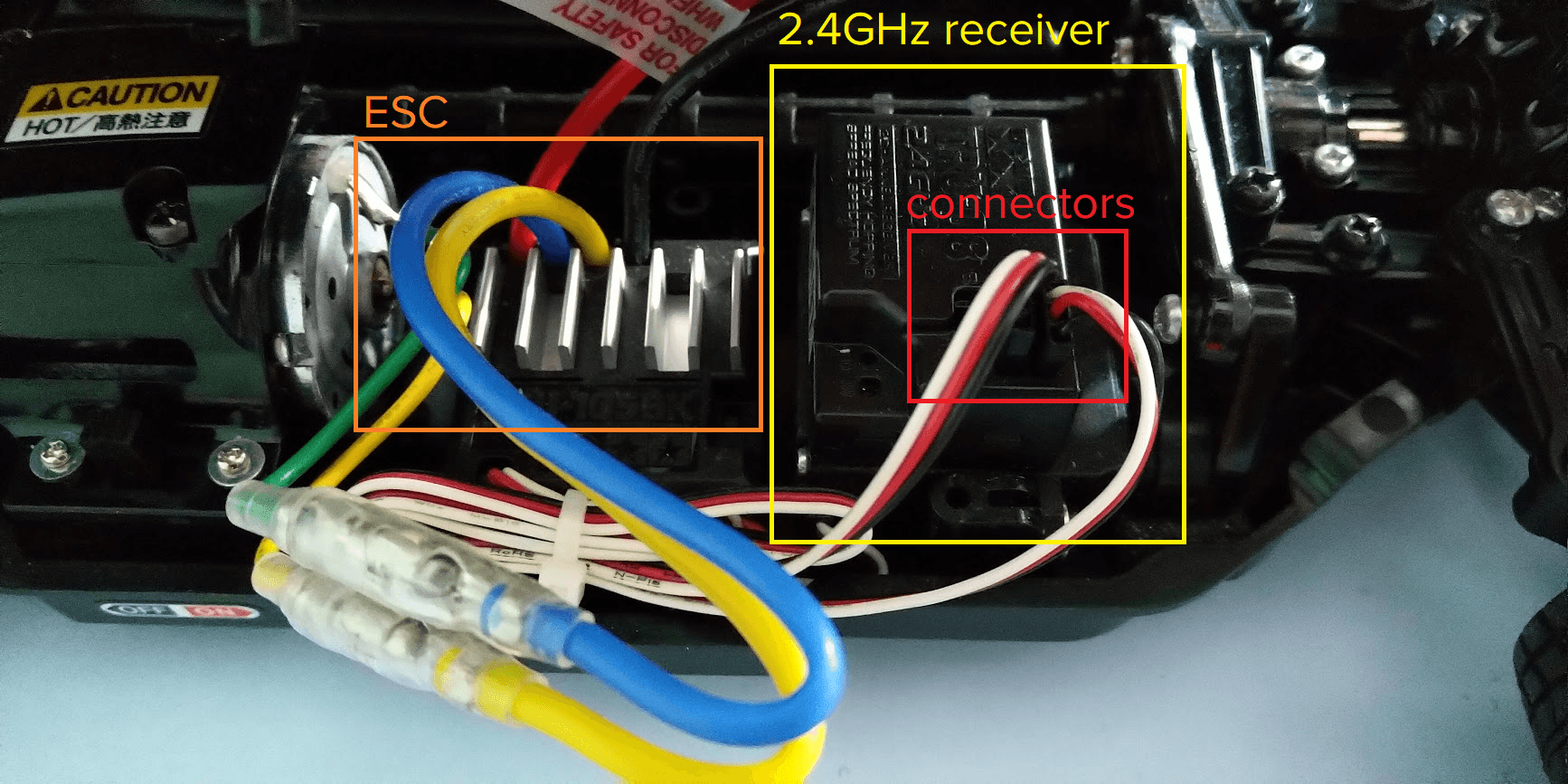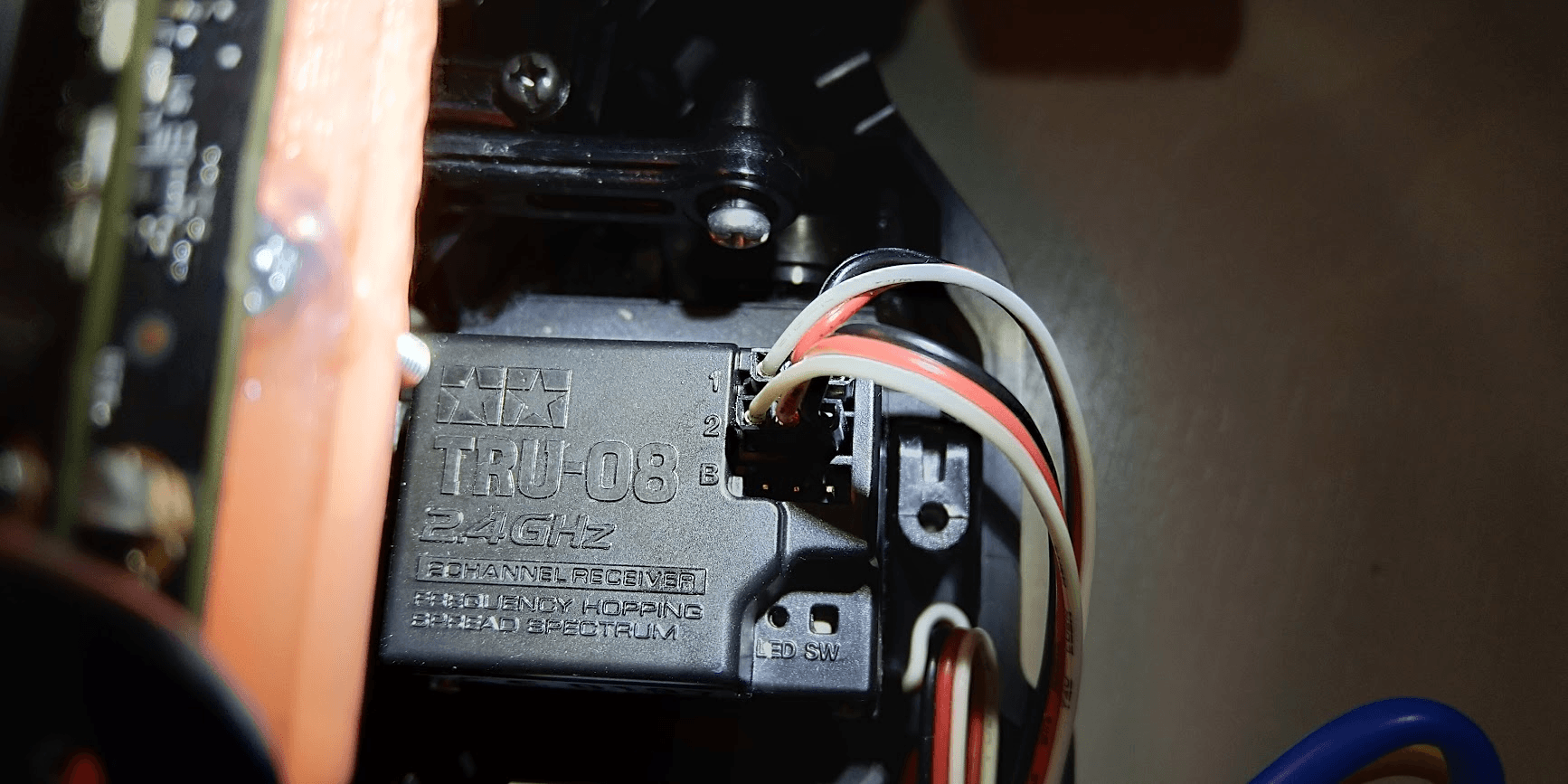### PCA9685### ESC -> PCA9685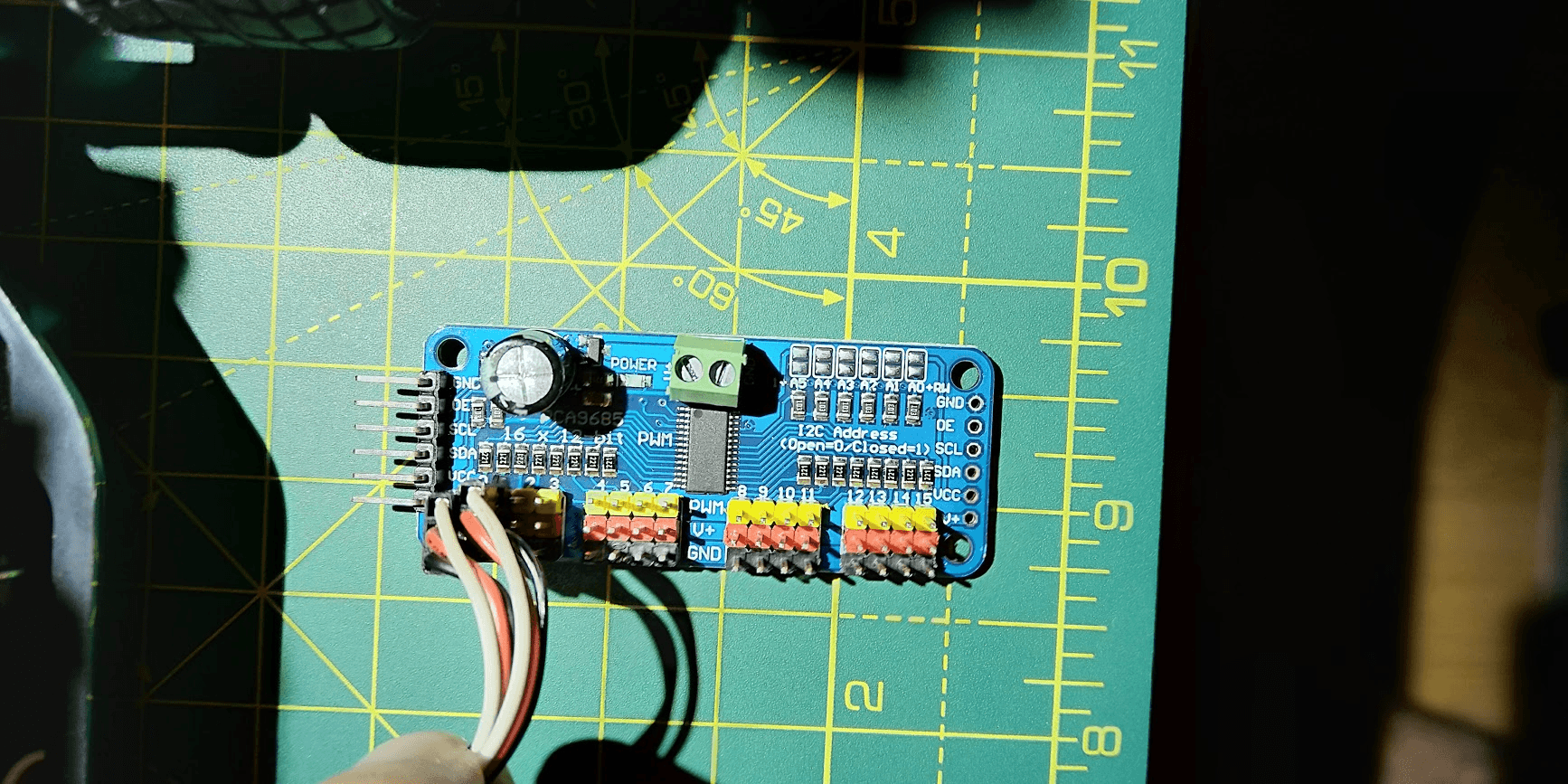### PCA9682 I2C Port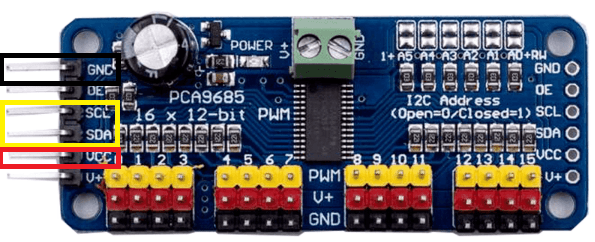### Jetson Nano I2C Pins### PCA9685 -> Jetson Nano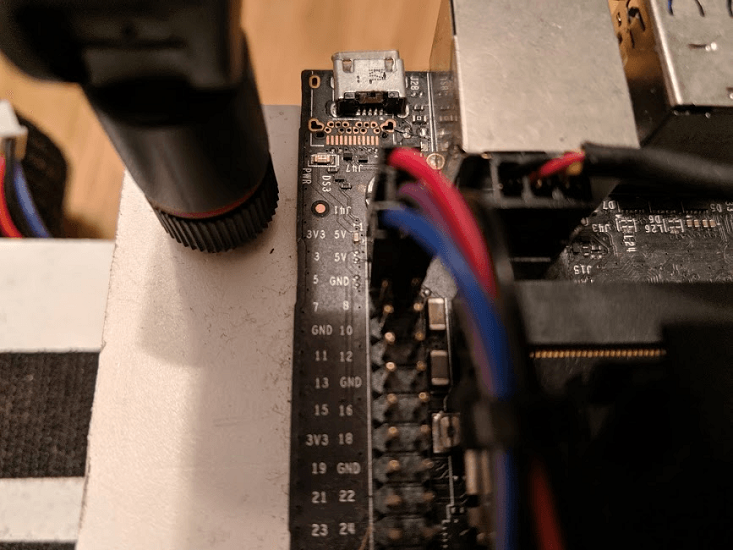## What is Donkey?

Donkey is a high level self driving library written in Python.

## How was it used?

It was used as an interface between the RC car and the neural net that controls the car.---

## Sanity check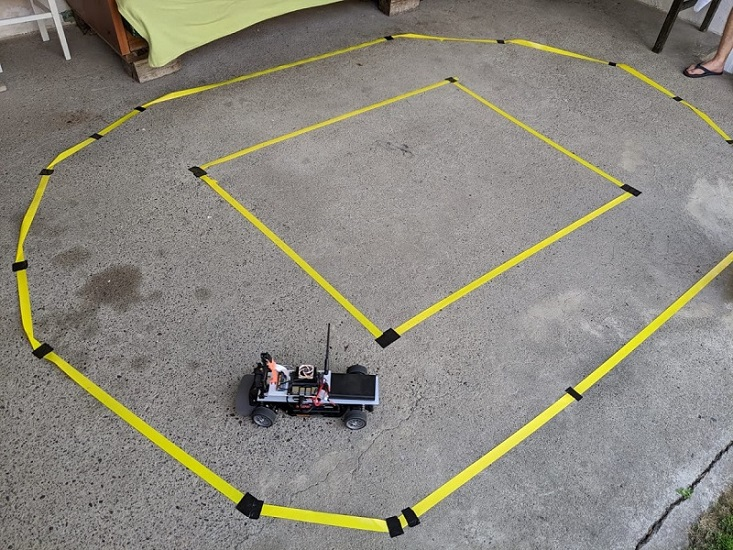## First test track## Fancier test track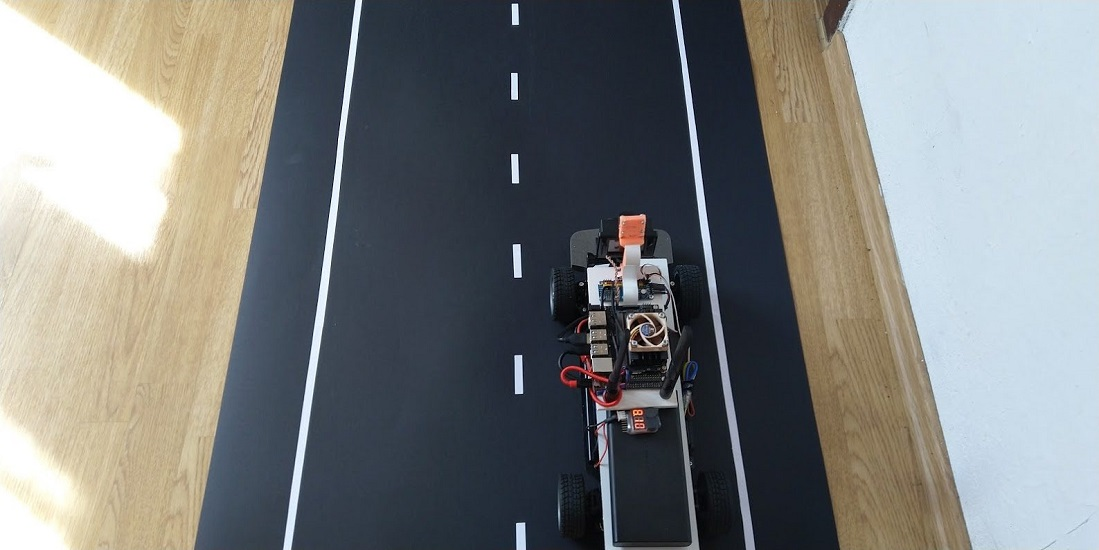## Why use a simulator?

Pretty simple: rapid prototyping in a safe and reproducible environment.

💡 Fun fact: Tesla spends a lot of time and money on their simulator.

Caveat emptor:

## Real-world fidelity

Physics? Model translation?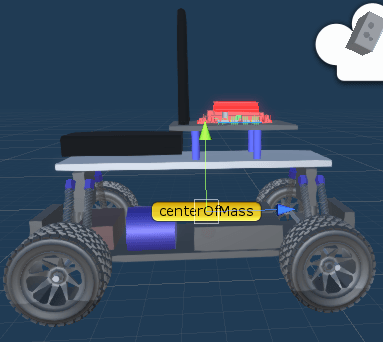## Dave

##### (DARPA Autonomous Vehicle)

An RC Car with two cameras autonomously driving through a junk-filled alley way.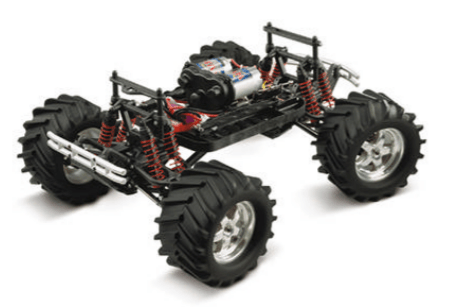## DAVE-2 Architecture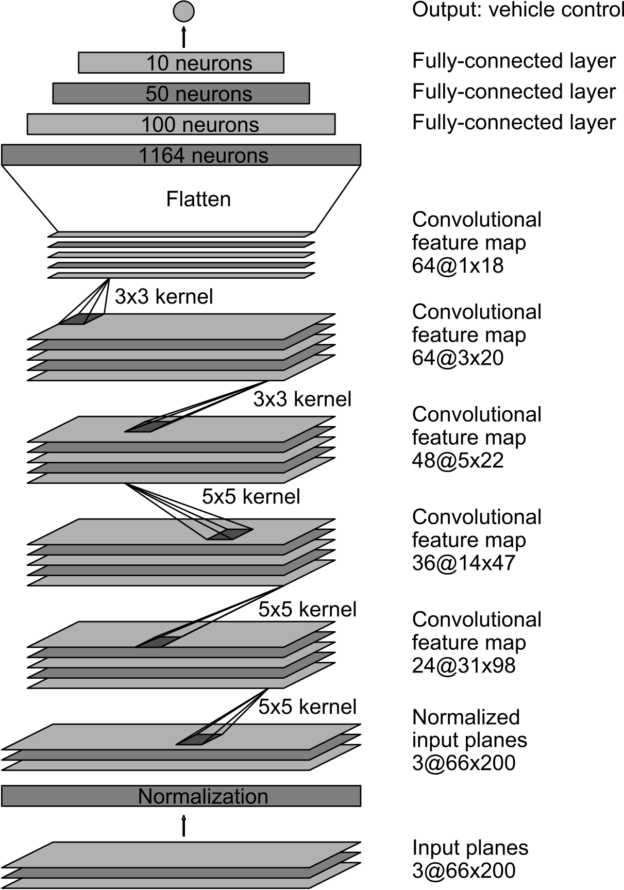## Implementing the DAVE-2 network

### Keras model

``````def customArchitecture(num_outputs, input_shape, roi_crop):

img_in = Input(shape=input_shape, name='img_in')
x = img_in

# Dropout rate
keep_prob = 0.9
rate = 1 - keep_prob

# Convolutional Layer 1
x = Convolution2D(filters=24, kernel_size=5, strides=(2, 2), input_shape = input_shape)(x)
x = Dropout(rate)(x)

# Convolutional Layer 2
x = Convolution2D(filters=36, kernel_size=5, strides=(2, 2), activation='relu')(x)
x = Dropout(rate)(x)

# Convolutional Layer 3
x = Convolution2D(filters=48, kernel_size=5, strides=(2, 2), activation='relu')(x)
x = Dropout(rate)(x)

# Convolutional Layer 4
x = Convolution2D(filters=64, kernel_size=3, strides=(1, 1), activation='relu')(x)
x = Dropout(rate)(x)

# Convolutional Layer 5
x = Convolution2D(filters=64, kernel_size=3, strides=(1, 1), activation='relu')(x)
x = Dropout(rate)(x)

# Flatten Layers
x = Flatten()(x)

# Fully Connected Layer 1
x = Dense(100, activation='relu')(x)

# Fully Connected Layer 2
x = Dense(50, activation='relu')(x)

# Fully Connected Layer 3
x = Dense(25, activation='relu')(x)

# Fully Connected Layer 4
x = Dense(10, activation='relu')(x)

# Fully Connected Layer 5
x = Dense(5, activation='relu')(x)
outputs = []

for i in range(num_outputs):
# Output layer
outputs.append(Dense(1, activation='linear', name='n_outputs' + str(i))(x))

model = Model(inputs=[img_in], outputs=outputs)

return model
``````

### Using the model in Donkey

``````class NvidiaModel(KerasPilot):
def __init__(self, num_outputs=2, input_shape=(120,160,3), roi_crop=(0,0), *args, **kwargs):
super(NvidiaModel, self).__init__(*args, **kwargs)
self.model = customArchitecture(num_outputs, input_shape, roi_crop)
self.compile()

def compile(self):
loss='mse')

def run(self, img_arr):
img_arr = img_arr.reshape((1,) + img_arr.shape)
outputs = self.model.predict(img_arr)
steering = outputs
throttle = outputs
return steering, throttle
``````

## “Hydra”-like nets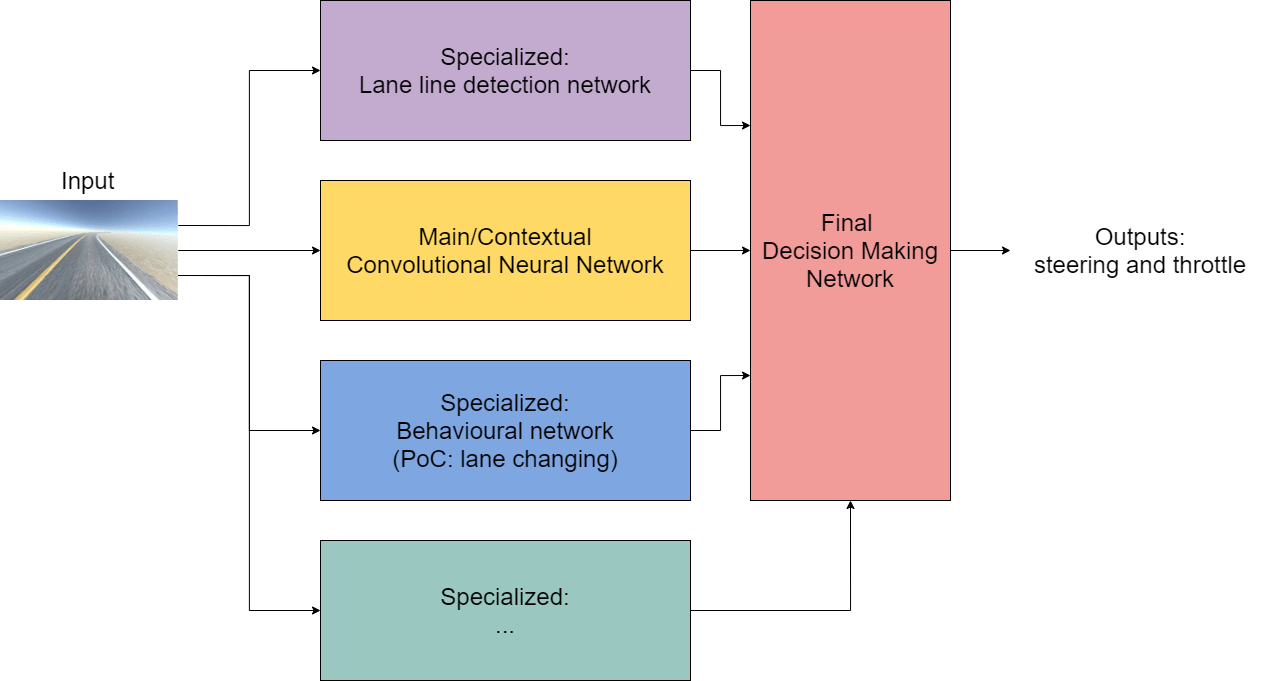## Tesla's similar nets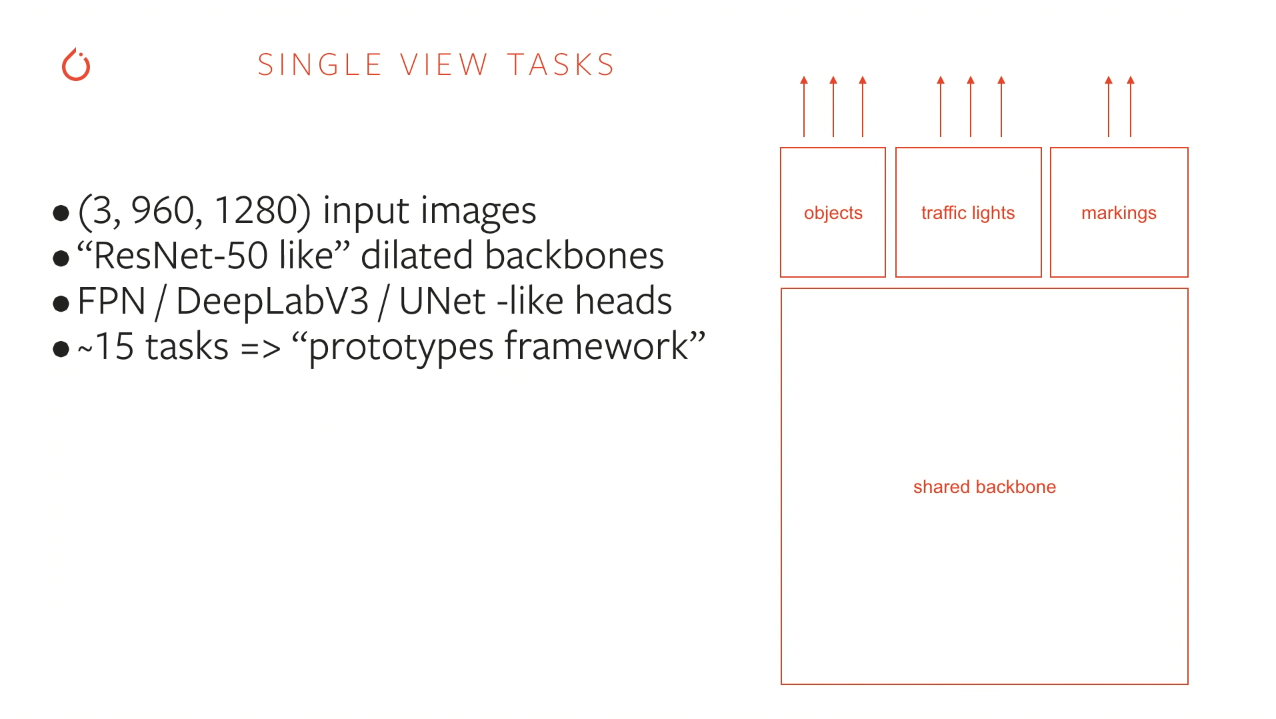## Mapping the 3D world to 2D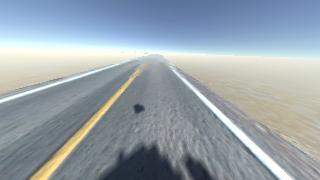## Parallel?## Another example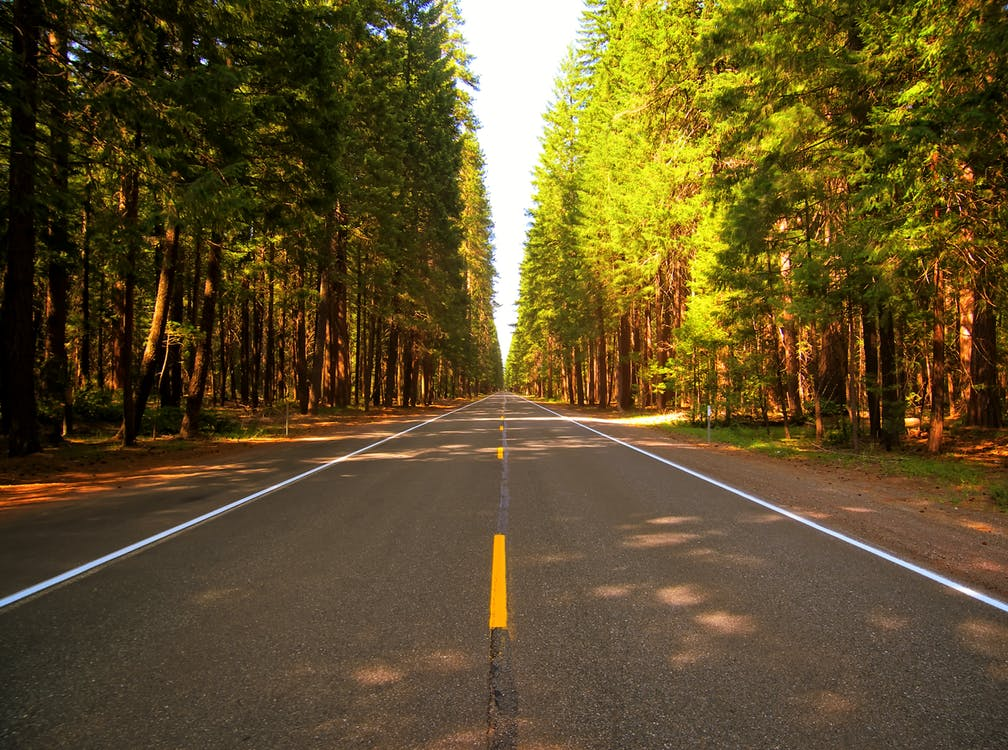## Parallel?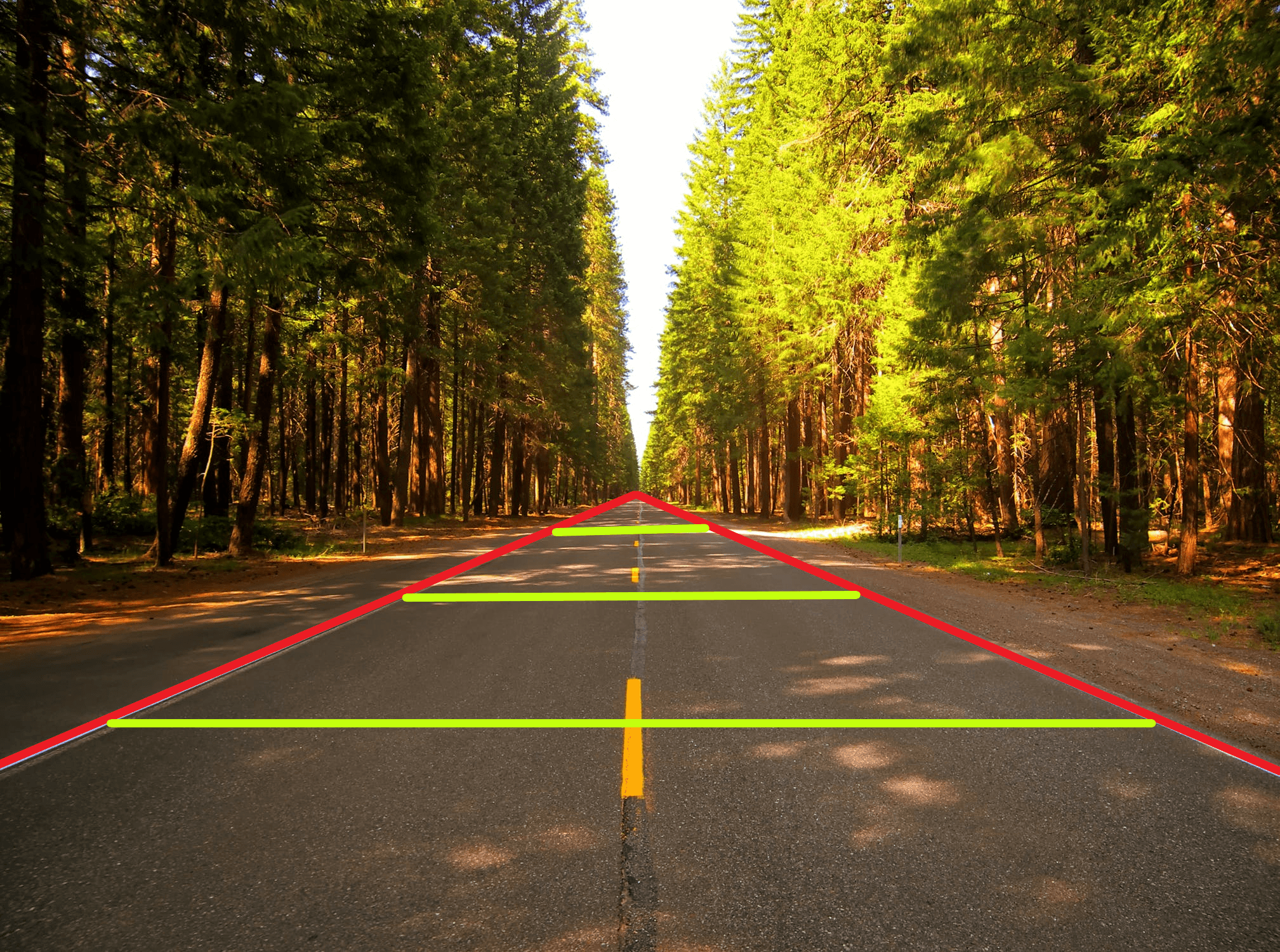## Train dronez?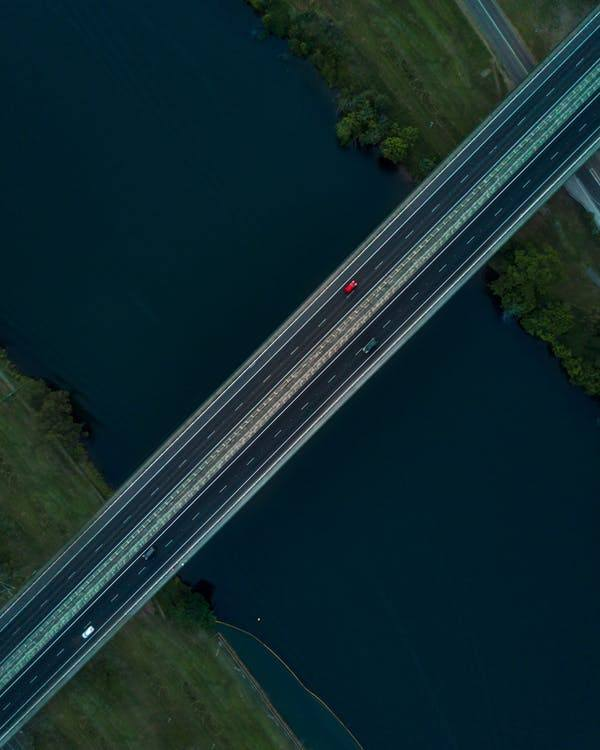## Warping the perspective## First step: ROI## Second step: target perspective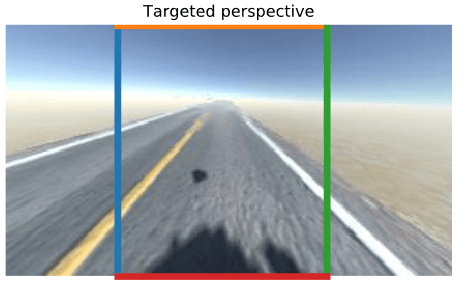## Final step: transform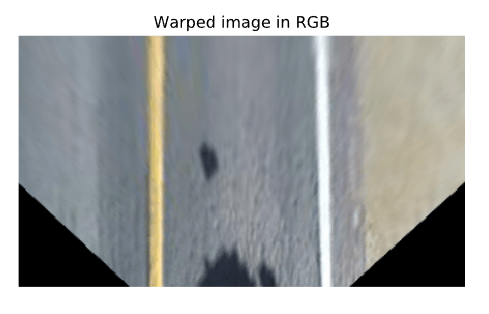## Converting to HSL color space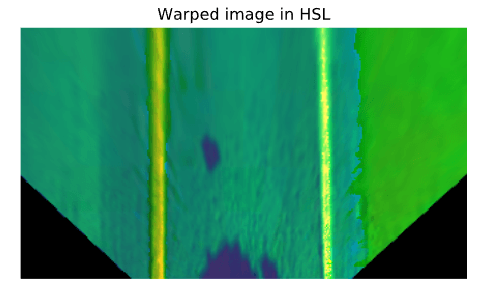## Split into separate channels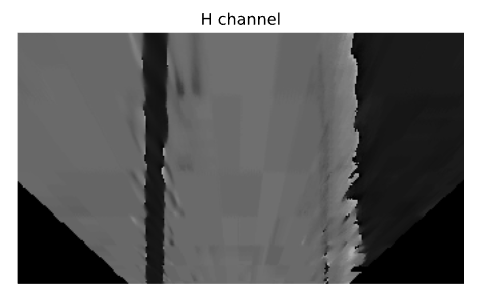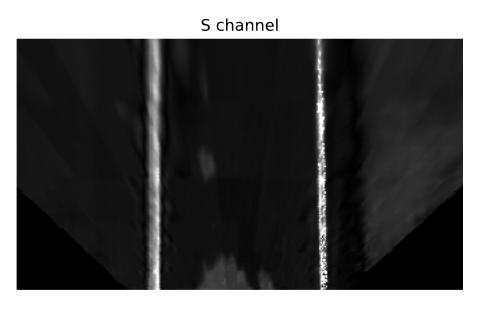## Threshold the S-channel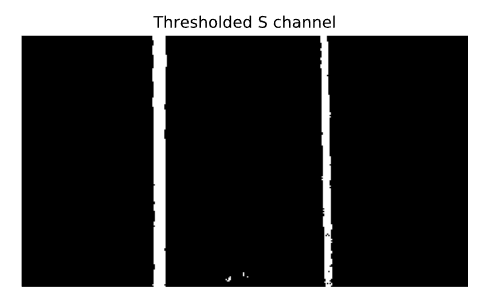## Going one step further?

### Fitting lanes using a polynomial approximation## Histogram overlay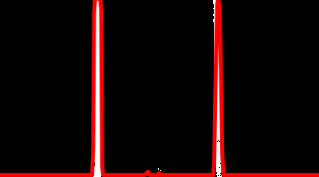### Found lane using polynomial fit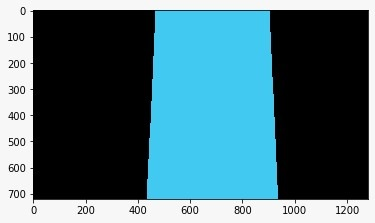### Unwarped image with detected lane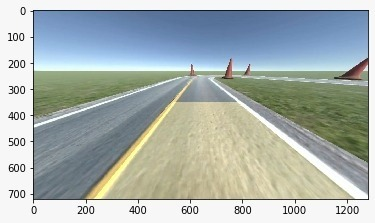## Why not to use it?

### Implementing the lane finding

``````def warpImage(self, image):
# Define the region of the image we're interested in transforming
regionOfInterest = np.float32(
[[0,  180],  # Bottom left
[112.5, 87.5], # Top left
[200, 87.5], # Top right
[307.5, 180]]) # Bottom right

# Define the destination coordinates for the perspective transform
newPerspective = np.float32(
[[80,  180],  # Bottom left
[80,    0.25],  # Top left
[230,   0.25],  # Top right
[230, 180]]) # Bottom right
# Compute the matrix that transforms the perspective
transformMatrix = cv2.getPerspectiveTransform(regionOfInterest, newPerspective)
# Warp the perspective - image.shape[:2] takes the height, width, [::-1] inverses it to width, height
warpedImage = cv2.warpPerspective(image, transformMatrix, image.shape[:2][::-1], flags=cv2.INTER_LINEAR)
return warpedImage

def extractLaneLinesFromSChannel(self, warpedImage):
# Convert to HSL
hslImage = cv2.cvtColor(warpedImage, cv2.COLOR_BGR2HLS)
# Split the image into three variables by the channels
hChannel, lChannel, sChannel = cv2.split(hslImage)
# Threshold the S channel image to select only the lines
lowerThreshold = 65
higherThreshold = 255
# Threshold the image, keeping only the pixels/values that are between lower and higher threshold
returnValue, binaryThresholdedImage = cv2.threshold(sChannel,lowerThreshold,higherThreshold,cv2.THRESH_BINARY)
# Since this is a binary image, we'll convert it to a 3-channel image so OpenCV can use it
thresholdedImage = cv2.cvtColor(binaryThresholdedImage, cv2.COLOR_GRAY2RGB)
return thresholdedImage

def processImage(self, image):
warpedImage = self.warpImage(image)
# We'll normalize it just to make sure it's between 0-255 before thresholding
warpedImage = cv2.normalize(warpedImage,None,0,255,cv2.NORM_MINMAX,cv2.CV_8U)
thresholdedImage = self.extractLaneLinesFromSChannel(warpedImage)
one_byte_scale = 1.0 / 255.0
# To make sure it's between 0-1 for the model
return np.array(thresholdedImage).astype(np.float32) * one_byte_scale
``````

## Implementing the first iteration model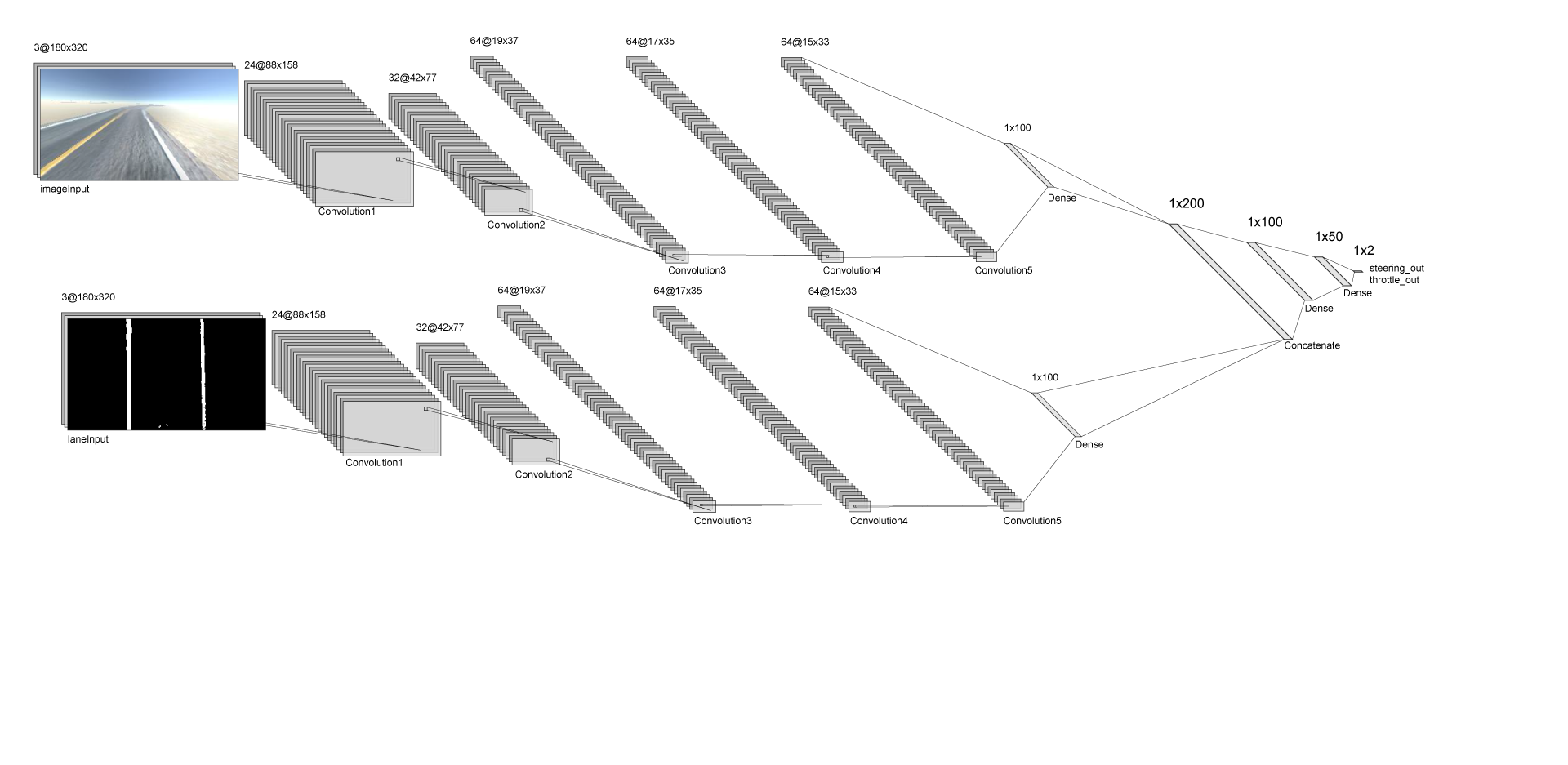## Model definition

``````def oriModel(inputShape, numberOfBehaviourInputs):

# Dropout rate
keep_prob = 0.9
rate = 1 - keep_prob

# Input layers
imageInput = Input(shape=inputShape, name='imageInput')
laneInput = Input(shape=inputShape, name='laneInput')
``````

## Generalized CNN

``````# Input image convnet
x = imageInput
x = Conv2D(24, (5,5), strides=(2,2), name="Conv2D_imageInput_1")(x)
x = LeakyReLU()(x)
x = Dropout(rate)(x)
x = Conv2D(32, (5,5), strides=(2,2), name="Conv2D_imageInput_2")(x)
x = LeakyReLU()(x)
x = Dropout(rate)(x)
x = Conv2D(64, (5,5), strides=(2,2), name="Conv2D_imageInput_3")(x)
x = LeakyReLU()(x)
x = Dropout(rate)(x)
x = Conv2D(64, (3,3), strides=(1,1), name="Conv2D_imageInput_4")(x)
x = LeakyReLU()(x)
x = Dropout(rate)(x)
x = Conv2D(64, (3,3), strides=(1,1), name="Conv2D_imageInput_5")(x)
x = LeakyReLU()(x)
x = Dropout(rate)(x)
x = Flatten(name="flattenedx")(x)
x = Dense(100)(x)
x = Dropout(rate)(x)
``````

## Lane finding CNN

``````# Preprocessed lane image input convnet
y = laneInput
y = Conv2D(24, (5,5), strides=(2,2), name="Conv2D_laneInput_1")(y)
y = LeakyReLU()(y)
y = Dropout(rate)(y)
y = Conv2D(32, (5,5), strides=(2,2), name="Conv2D_laneInput_2")(y)
y = LeakyReLU()(y)
y = Dropout(rate)(y)
y = Conv2D(64, (5,5), strides=(2,2), name="Conv2D_laneInput_3")(y)
y = LeakyReLU()(y)
y = Dropout(rate)(y)
y = Conv2D(64, (3,3), strides=(1,1), name="Conv2D_laneInput_4")(y)
y = LeakyReLU()(y)
y = Dropout(rate)(y)
y = Conv2D(64, (3,3), strides=(1,1), name="Conv2D_laneInput_5")(y)
y = LeakyReLU()(y)
y = Flatten(name="flattenedy")(y)
y = Dense(100)(y)
y = Dropout(rate)(y)
``````

## Concatenating the CNNs

``````# Concatenated final convnet
c = Concatenate(axis=1)([x, y])
c = Dense(100, activation='relu')(c)
c = Dense(50, activation='relu')(c)
``````

## Outputs

``````# Output layers
steering_out = Dense(1, activation='linear', name='steering_out')(o)
throttle_out = Dense(1, activation='linear', name='throttle_out')(o)
model = Model(inputs=[imageInput, laneInput, behaviourInput], outputs=[steering_out, throttle_out])

return model
``````

## Training results

• 10k records made on the randomly generated track
• 12 epochs, 21m 45s on an RTX 2060
• The final validation loss was 0.003665.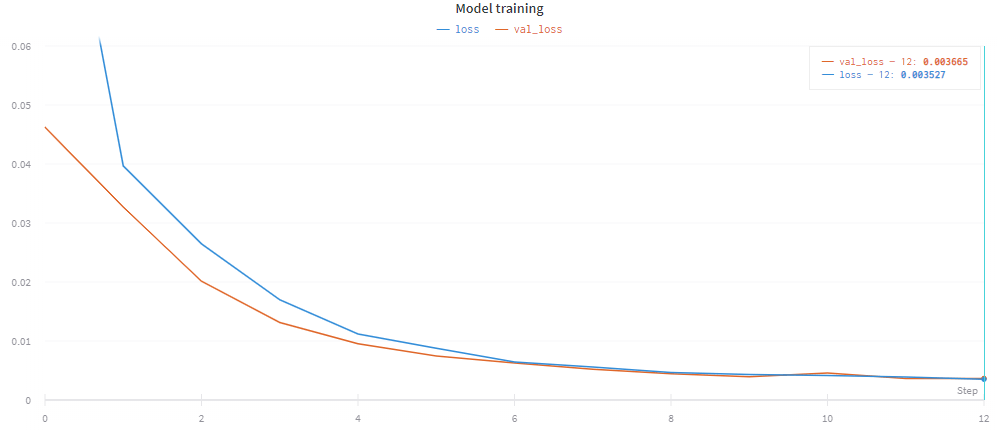## The architecture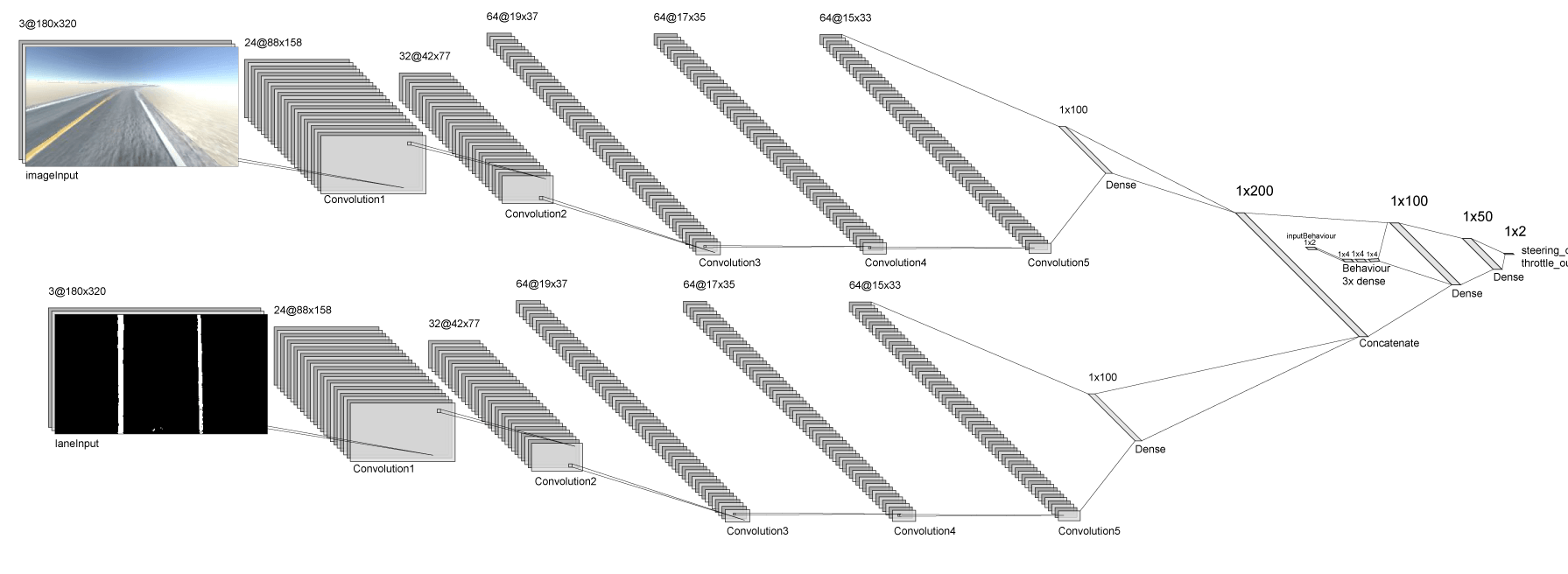## Implementation

``````# New input layer
behaviourInput = Input(shape=(numberOfBehaviourInputs,), name="behaviourInput")

# ConvNet parts ...

# Behavioural net
z = behaviourInput
z = Dense(numberOfBehaviourInputs * 2, activation='relu')(z)
z = Dense(numberOfBehaviourInputs * 2, activation='relu')(z)
z = Dense(numberOfBehaviourInputs * 2, activation='relu')(z)

# Concatenating the convolutional networks with the behavioural network
o = Concatenate(axis=1)([z, c])
o = Dense(100, activation='relu')(o)
o = Dense(50, activation='relu')(o)

# Output layers ...

# Update the model inputs
model = Model(inputs=[imageInput, laneInput, behaviourInput], outputs=[steering_out, throttle_out])
``````

## Training

• 7k records with approx. 20 lane changes
• Final validation loss: 0.003947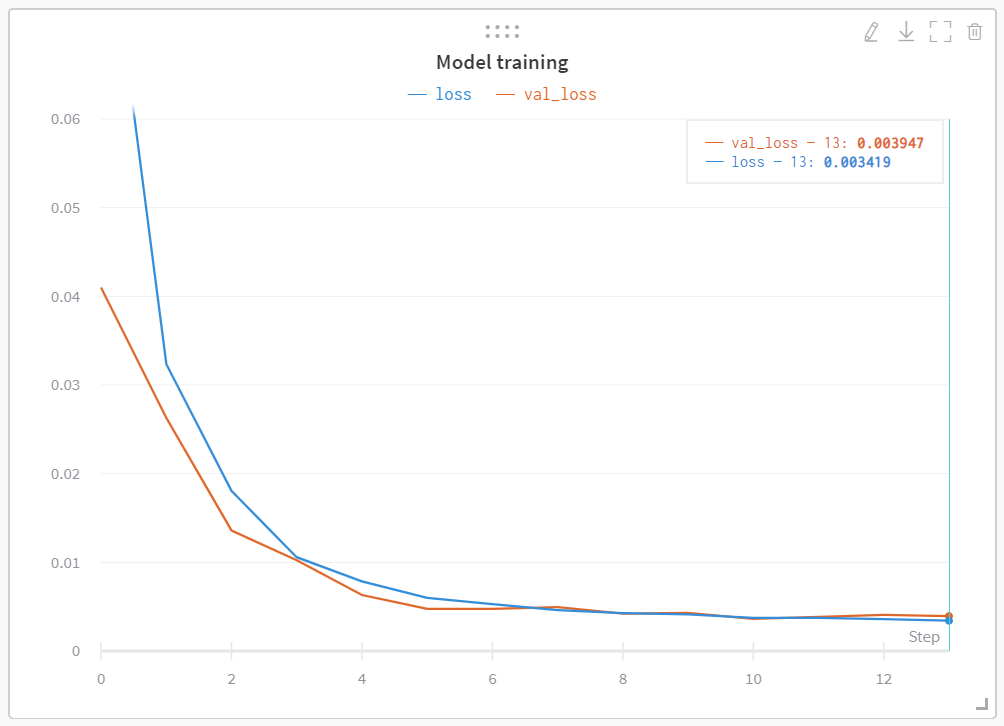## Further avenues

### Advanced lane finding with object recognition